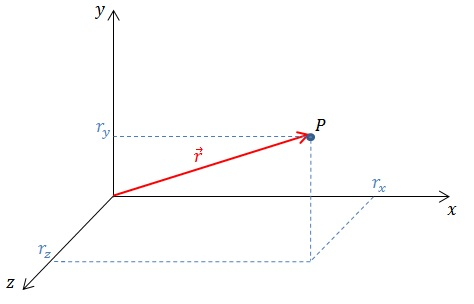# How to Calculate Displacement

Before learning to calculate displacement, let us define displacement and learn what is position vector and how to write it.

## Definition of Displacement

Displacement is the measurement of the difference between a particle’s initial and final position. It is one of the basic quantities used in kinematics, which is also used to derive velocity and acceleration. Displacement is a vector quantity, which has both a magnitude (size) and a direction. To calculate displacement, you need to subtract the position vector of the initial position from the position vector of the final position. So, before discussing displacement, it is important to understand how a position is denoted using vectors.

## What is Position Vector

position vector gives the position of a particle with respect to the origin of the coordinate system. In our discussion, we will limit ourselves to a system of 3-dimensional Cartesian coordinates. The position vector$\vec r$ for a particle$P$ at coordinates$(r_x, r_y, r_z)$$\vec r = (r_x, r_y, r_z)$.## How to Calculate Displacement

Suppose a particle moves from a point$P_1$, that has a position vector$\vec{r_1} = (r_{1x}, r_{1y}, r_{1z})$ to a new position$P_2$ with a position vector position vector$\vec{r_2} = (r_{2x}, r_{2y}, r_{2z})$. Then the displacement vector$\vec{\Delta r}$ is given by$\vec{\Delta r} = \vec{r_2} - \vec{r_1} = (r_{2x}-r_{1x}, r_{2y}-r_{1y}, r_{2z}-r_{1z})$.Example

A particle moves from position$(3, -2, 0)$ to$(5, -8, -4)$. Calculate displacement vector for this motion.

We have$\vec{r_1} = (3, -2, 0)$ and$\vec{r_2} = (5, -8, -4)$. Therefore,$\vec{\Delta r} = (5-3, -8-(-2), -4-0) = (2, -6, -4)$.

## How to Calculate Net Displacement

Suppose a particle moves several times. The net displacement is the displacement vector between the particle’s initial position and the final position. The net displacement can also be obtained by vector addition of each of the individual displacement vectors that corresponds to each stage of motion. For example, in the diagram below, the position vector of the point$P_1$ is$\vec{r_1}$ and the position vector of  the point$P_4$ is$\vec{r_4}$. Then, the net displacement$\vec{r_{net}} = \vec{r_4} - \vec{r_1} = \vec{\Delta r_1} + \vec{\Delta r_2} + \vec{\Delta r_3}$.## How to Calculate Magnitude of Displacement

As mentioned earlier, displacement is a vector quantity. The magnitude (size) of this vector quantity gives the distance. If the displacement vector$\vec{\Delta r} = (r_x, r_y, r_z)$, then the magnitude$\left |\vec{r}\right |$ is given by$\left |\vec{r}\right | = \sqrt{{r_x}^{2} + {r_y}^{2} + {r_z}^{2}}$.

In the earlier example, we had a displacement vector of$(2, -6, -4)$. The magnitude of this vector is$\left |\overrightarrow{r}\right | = \sqrt{{2}^{2} + {(-6)}^{2} + {(-4)}^{2}} = \sqrt{56} \approx 7.5 \textup{ units}$.